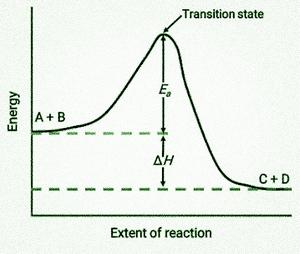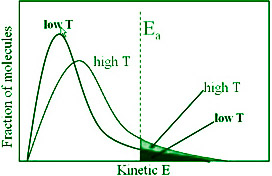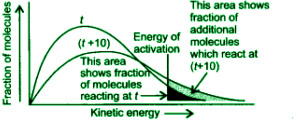GFG App
Open AppBrowser
Continue

# Temperature Dependence of the Rate of a Reaction

The meal cooks slowly if the gas is kept at a low temperature while cooking. When we raise the temperature to its highest setting, however, the food cooks quickly. As a result, increasing the temperature increases the rate of a reaction. The Arrhenius equation helps explain this rate-temperature relationship. Let’s have a look at this equation and see how it works.

### Temperature dependence of the rate of a reaction

Activation energy comes into play. A reaction occurs when the reactant molecules clash with each other, according to collision theory. The threshold energy is the smallest amount of energy that colliding molecules must have in order for their collision to be effective.

The activation energy is the lowest additional amount of energy absorbed by the reactant molecules in order for their energy to equal the threshold value.

Threshold energy = Activation energy + Energy possessed.

The lower the activation energy, the faster the reaction. The reactants must overcome an energy barrier in order to transform into products. Reactant molecules absorb energy and create an intermediate called an activated complex, which dissociates into the products almost instantly.From left to right, the diagram is viewed. At first, the system contains solely reactants (A + B). When sufficient energy reactant molecules collide, they generate a high-energy activated complex or transition state. After that, the unstable transition state decays to produce stable products (C + D).

The activation energy of the reaction, Ea, is shown as the energy difference between the reactants and the transition state in the diagram. The enthalpy change of the reaction (H) is equal to the energy difference between the reactants and products. The reaction is exothermic (H<0) in this example because it results in a drop in the system enthalpy.

#### Temperature Dependence of the rate of a reaction

The rate constant approximately doubles for a chemical process when the temperature rises by ten degrees.

Temperature coefficient = Rate constant at T + 10° / Rate constant at T°

Explanation

If fractions of molecules are plotted against corresponding kinetic energies at a specific temperature, a graph similar to the one depicted is created. The most probable kinetic energy is represented by the peak of the curve, which indicates the kinetic energy possessed by the largest fraction of molecules.

With the increase in temperature

• The maximum of the curve shifts to a higher energy value, indicating that the most likely kinetic energy increases.
• The curve shifts to the right, indicating that there are more molecules with very high energies.

Since total probability must always be one, the area under the curve remains constant. At (t + 10), the region depicting the fraction of molecules with energy equal to or greater than activation energy doubles, resulting in a reaction rate doubled.

### Effect of temperature

Temperature is one of the variables that have a significant impact on the rate of a chemical reaction. Milk has frequently been spotted boiling on a gas stove. The rate at which a certain amount of milk boils is determined by the stove’s flame. The milk boils faster if the flame height is set to maximum, while it takes longer if the flame height is set to minimum. The height of the flame here corresponds to the temperature.

When the temperature is high, the milk boils faster, and when the temperature is low, the milk takes longer to boil. The temperature has an impact on many reactions, including the boiling of milk. The reaction rate of the majority of chemical reactions changes as the temperature changes.

For every 10 degrees Celsius increase in temperature, the rate constant for a chemical reaction doubles. There were no definite means to physically measure the temperature dependency of a chemical reaction’s pace until 1889. Svante Arrhenius improved J.H van’t Hoff’s work in 1889 by proposing an equation that quantitatively connected temperature and the rate constant for a process. Arrhenius Equation was the name given to the proposed equation.

### Arrhenius equation

The Arrhenius equation can quantitatively explain the temperature dependency of the rate of a chemical process.

k = Ae-Ea/RT

The Arrhenius factor, also known as the frequency factor or pre-exponential factor, is represented by A. Ea is the activation energy in joules/mole, and R is the gas constant.The fraction of molecules with kinetic energy larger than Ea is represented by the factor e-Ea/RT.

As a result of the Arrhenius equation, it has been discovered that increasing the temperature or decreasing the activation energy causes an increase in the reaction rate and an exponential increase in the rate constant.Taking both sides of the equation’s natural logarithm

ln k = -(Ea/RT) + ln A

A straight line with slope is drawn when ln k vs 1/T is plotted = -(Ea/R) and intercept = ln A

At temperature T1, equation

ln k1 = Ea/RT1 + ln A

At temperature T2, equation

ln k2 = Ea/RT2 + ln A

For a given reaction, A is constant.

The values of rate constants for temperatures T1 and T2 are k1 and k2, respectively.

Subtracting equation form,

ln k2 – ln k1 = (Ea/RT1) – (Ea/RT2)

ln (k2/k1) = Ea/R ((1/T1)-(1/T2))

log k2/k1 = (Ea/2.303R) × ((1/T1)-(1/T2))

log k2/k1 = (Ea/2.303R) × ((T2-T1)/(T1T2))

### Graphical description of effect of temperature

The average kinetic energy of molecules is proportional to temperature, it has been discovered. A bimolecular reaction, according to the collision theory, occurs only when the reacting molecules collide with adequate kinetic energy and suitable orientation.

The fraction of molecules with kinetic energy equal to or greater than Ea at a given temperature may lead to the product. As the temperature rises, the proportion of molecules with energies equal to or greater than (>= Ea) increases. As a result, the reaction rate would increase. Plotting a fraction of molecules with particular kinetic energy versus kinetic energy for two distinct temperatures T1 and T2 illustrates this.The number of molecules having those levels of kinetic energy is proportional to the area under the curve. At T1 and T2, the entire area is the same. The fraction of molecules with kinetic energy greater than Ea at T1 and T2 are represented by the areas (a) and (b). This means that when the temperature rises, the percentage of molecules having energies greater than Ea rises. As a result, the velocity of the reaction quickens.

### Effect of Catalyst

A catalyst is a material that enhances the rate of a reaction while not undergoing any lasting chemical changes. Intermediate Complex Idea: A catalyst makes transient bonds with reactants to produce an intermediate complex, according to this theory. This complex decomposes over time, releasing products and the catalyst.

A catalyst decreases the potential energy barrier by lowering the activation energy. As a result, the catalyst gives an alternative reaction pathway. Catalyze a large number of reactants with a minimal amount of catalyst. Gibbs’ energy is not changed by a catalyst. A catalyst can only catalyze spontaneous reactions and not non-spontaneous reactions. Furthermore, a catalyst does not alter the equilibrium constant; rather, it aids in the speedier attainment of equilibrium.

### Sample Problems

Question 1: In chemicals, what is the reaction rate?

The frequency with which a chemical reaction occurs is referred to as reaction rate in chemistry. It can also be described in terms of the concentration of a material generated in a time unit (amount per unit volume) or the concentration of a reactant absorbed in a time unit.

Question 2: How does an enzyme speed up a reaction?

Enzymes are enzymes that act as biochemical catalysts. Catalysts reduce the amount of energy required to initiate reactions. The activation energy of a reaction decreases as the rate of the reaction increases. Enzymes also accelerate processes by lowering the activation energy.

Question 3: Explain Temperature Dependence of the rate of a reaction.

The rate constant approximately doubles for a chemical process when the temperature rises by ten degrees.

Temperature coefficient = Rate constant at T + 10° / Rate constant at T°

Explanation:

If fractions of molecules are plotted against corresponding kinetic energies at a specific temperature, a graph similar to the one depicted is created. The most probable kinetic energy is represented by the peak of the curve, which indicates the kinetic energy possessed by the largest fraction of molecules.

With increase in temperature:

The maximum of the curve shifts to a higher energy value, indicating that the most likely kinetic energy increases. The curve shifts to the right, indicating that there are more molecules with very high energies.

Since total probability must always be one, the area under the curve remains constant. At (t +10), the region depicting the fraction of molecules with energy equal to or greater than activation energy doubles, resulting in a reaction rate doubled.

Question 4: Define the Effect of temperature.

Temperature is one of the variables that has a significant impact on the rate of a chemical reaction. Milk has frequently been spotted boiling on a gas stove. The rate at which a certain amount of milk boils is determined by the stove’s flame. The milk boils faster if the flame height is set to maximum, while it takes longer if the flame height is set to minimum. The height of the flame here corresponds to the temperature.

When the temperature is high, the milk boils faster, and when the temperature is low, the milk takes longer to boil. Temperature has an impact on many reactions, including the boiling of milk. The reaction rate of the majority of chemical reactions changes as the temperature changes.

For every 10° Celsius increase in temperature, the rate constant for a chemical reaction doubles. There was no definite means to physically measure the temperature dependency of a chemical reaction’s pace until 1889. Svante Arrhenius improved J.H van’t Hoff’s work in 1889 by proposing an equation that quantitatively connected temperature and the rate constant for a process. Arrhenius Equation was the name given to the proposed equation.

Question 5: What role does pH play in reaction rate?

Chemical reactions can be speed up or slowed down by changing the pH, temperature, or concentration of the substratum. The enzyme complex to which it interacts is referred to as the substrate. The enzyme’s reaction rate increases at appropriate pH, but decreases at less than optimal pH. It is eventually harmed when an enzyme is denatured.

Question 6: Explain the Effect of the Catalyst in brief.

A catalyst is a material that enhances the rate of a reaction while not undergoing any lasting chemical changes. Intermediate Complex Idea: A catalyst makes transient bonds with reactants to produce an intermediate complex, according to this theory. This complex decomposes over time, releasing products and the catalyst.

A catalyst decreases the potential energy barrier by lowering the activation energy. As a result, the catalyst gives an alternative reaction pathway. Catalyze a large number of reactants with a minimal amount of catalyst. Gibbs energy is not changed by a catalyst. A catalyst can only catalyze spontaneous reactions and not non-spontaneous reactions. Furthermore, a catalyst does not alter the equilibrium constant; rather, it aids in the speedier attainment of equilibrium.

Question 7: Write the Arrhenius equation and explain the terms involved in it.

k = Ae-Ea/RT

Where,

k = rate constant of the reaction

A = Arrhenius Constant

Ea = Activation Energy for the reaction (in Joules mol-1)

R = Universal Gas Constant

T = Temperature in absolute scale (in kelvins)

My Personal Notes arrow_drop_up